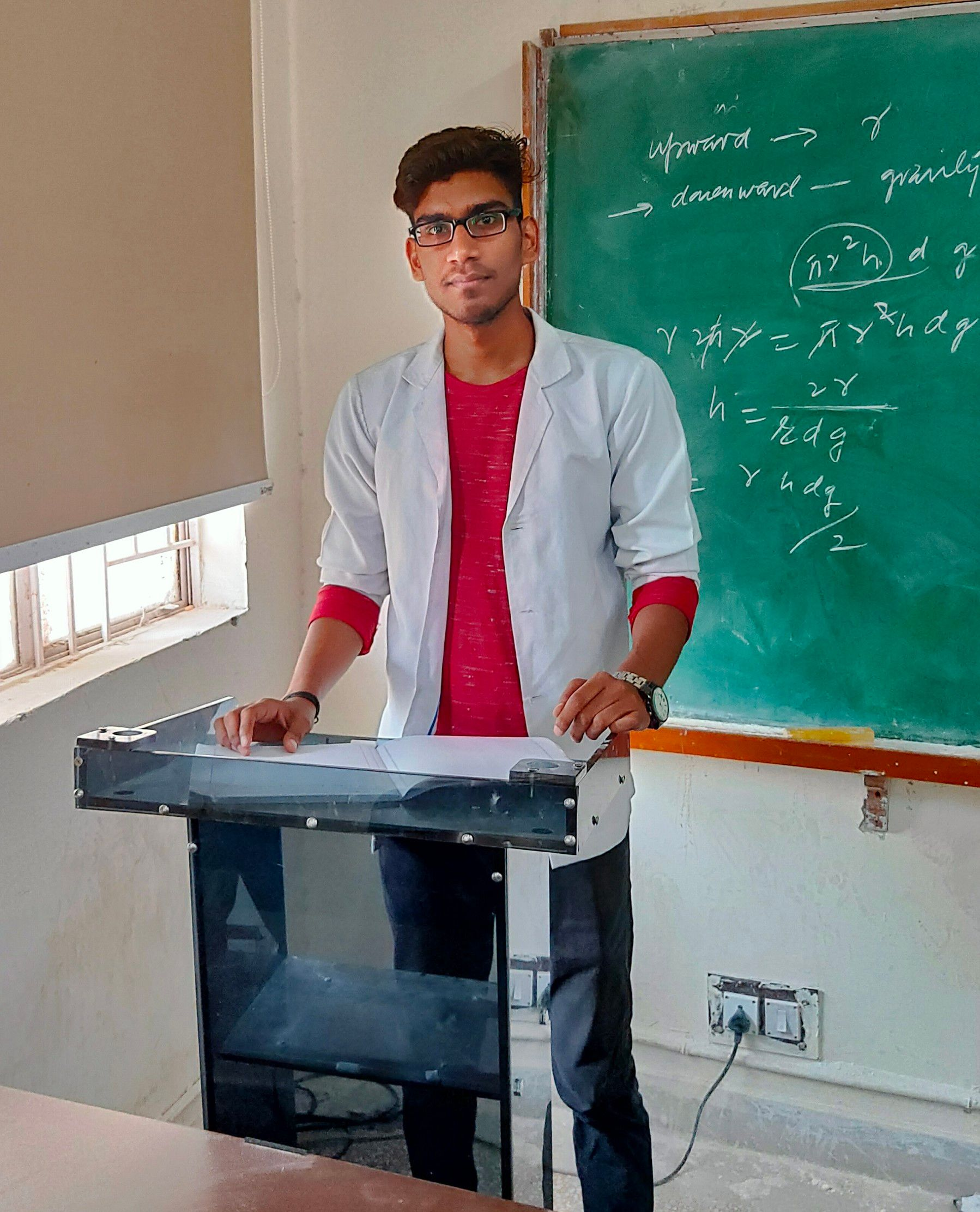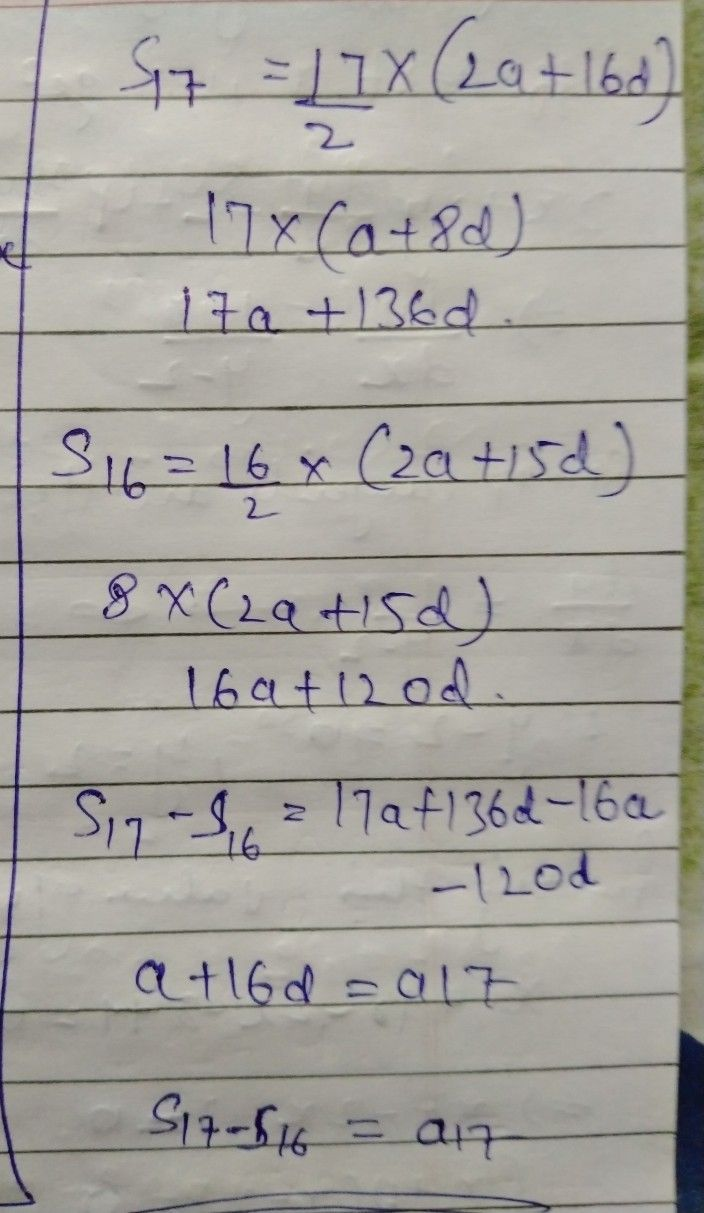Symbol
Problem$-A$ ATTHMETIC PROGRESSIONS $s_{17}-S_{16}=7$ $\left(a\right)$ $S_{1}$ $\left(b\right)$ $T_{1}$ $\left(c\right)$ $T_{17}$ $\left(d\right)$
10th-13th grade
Other
SolutionQanda teacher - Gulshan123for further queries you can contact me...Student
thank you